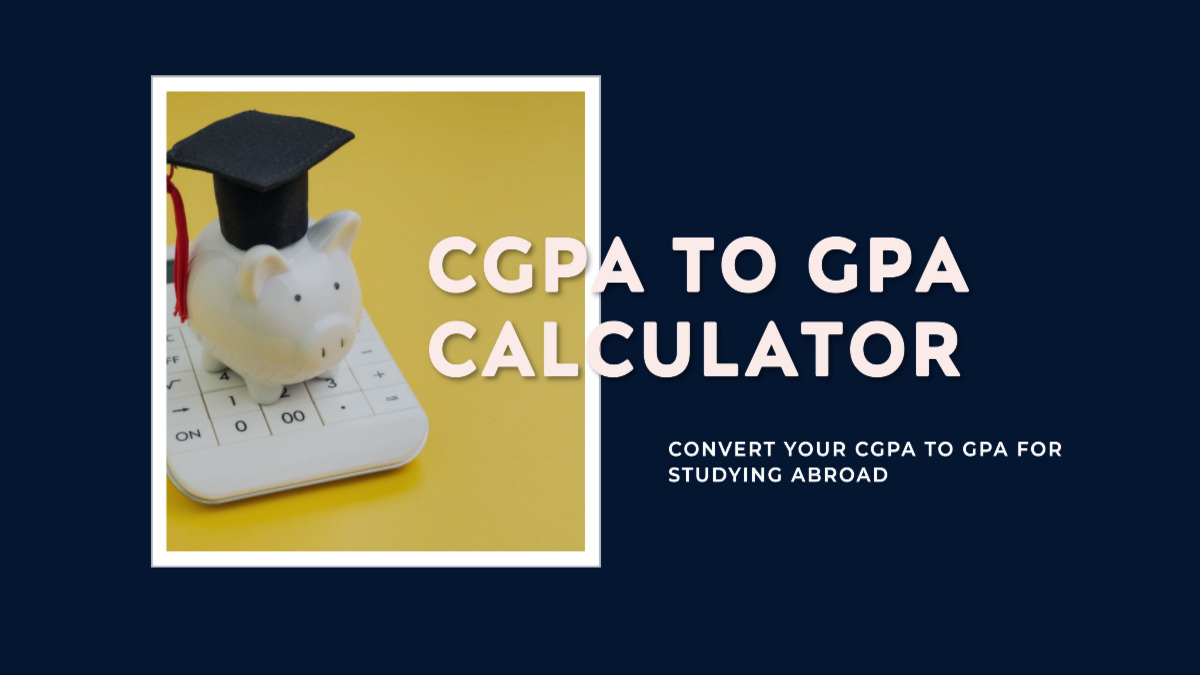## Converting CGPA to GPA for Studying Abroad: Calculator IncludedConverting CGPA to GPA for Studying Abroad: Calculator Included
• Post author:
• Post category:Education

CGPA to GPA Calculator : Converting 10-Point CGPA to 4-Point GPA for Studying Abroad: Calculator Included

Understand the process of converting a 10-point CGPA to a 4-point GPA for studying abroad. Learn the significance of GPA and CGPA, the conversion formula, and use our calculator for quick results.

## Introduction

For students aiming to pursue higher education abroad, understanding the conversion between different grading systems is crucial. In this blog post, we will explore the process of converting a 10-point Cumulative Grade Point Average (CGPA) to a 4-point Grade Point Average (GPA), specifically tailored for Indian students seeking admission in universities abroad. We will delve into the significance of GPA and CGPA, the conversion process, and provide a handy reference table for quick estimation.

## What is GPA and CGPA?

GPA, or Grade Point Average, is a numerical representation of a student’s academic performance in a single semester or term. It is calculated by averaging the grades obtained in all the courses taken during that term. On the other hand, CGPA, or Cumulative Grade Point Average, provides an overall assessment of a student’s performance throughout their entire academic journey, considering grades from all semesters.

## Converting 10-Point CGPA to 4-Point GPA

The conversion process from a 10-point CGPA to a 4-point GPA involves scaling down the CGPA to fit the different grading system. While universities generally handle this conversion, students can estimate their GPA for reference using a simple formula:

### GPA = (CGPA / Maximum CGPA) * 4

For instance, if your CGPA is 7.0 out of a maximum 10.0 CGPA, the conversion would be:

### Using the Calculator

We’ve made things even simpler with our CGPA to GPA calculator. Just follow these steps:

1. Enter your 10-point CGPA in the input field provided.
2. Click the “Convert” button.
3. The calculator will instantly display your equivalent 4-point GPA.

CGPA to GPA Calculator with Grade Equivalent

## CGPA to GPA Calculator with Grade Equivalent

Enter your 10-point CGPA to calculate the equivalent 4-point GPA and grade equivalent:

### Reference Table for Conversion

Here’s a quick reference table to estimate your 4-point GPA based on your 10-point CGPA:

Note: This table offers a rough approximation and may vary slightly between universities.

## Conclusion

Converting a 10-point CGPA to a 4-point GPA is essential when applying to universities abroad. While institutions typically manage the conversion process, having a basic understanding allows students to assess their academic standing accurately. Remember, the conversion may vary, so it’s always wise to refer to official guidelines or consult university authorities for precise conversions.

### How to convert CGPA to GPA?

Divide your CGPA by the maximum possible CGPA (usually 10) and then multiply by 4 to get the equivalent GPA on a 4.0 scale.

### Is CGPA the same as GPA?

While GPA represents a single semester’s performance, CGPA considers cumulative performance over all semesters.

### Which organizations provide credential evaluations for international students?

Organizations like World Education Services (WES) and Educational Perspectives (EP) offer credential evaluation services to make academic documents internationally recognized.